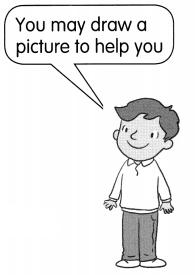Practice the problems of Math in Focus Grade 1 Workbook Answer Key Chapter 9 Length to score better marks in the exam.

Math Journal

Help Jamie put his toys away. Read. Then cut out the toys on page 227 and paste them on the shelf.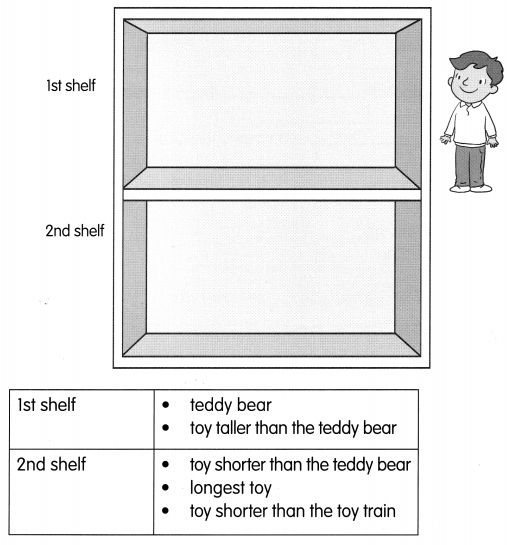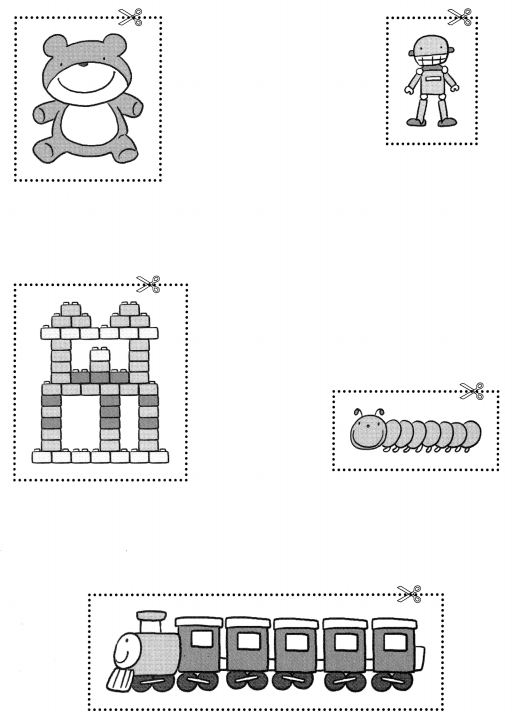Challenging Practice

Solve.

Mae moves the counters on a board. The arrows show the moves.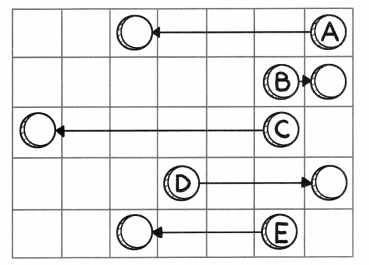Question 1.
Which counter makes the longest move?
__________
Answer: Counter C makes the longest move.

Question 2.
Which counter makes the shortest move?
__________
Answer: Counter B makes the shortest move.

Question 3.
Which counter moves 5 squares? __________
Answer: Counter C moves 5 squares.

Question 4.
Which counters move the same length? ___ and ___
Answer: D and E counters moves the same length.

Three boys are lying on a mat.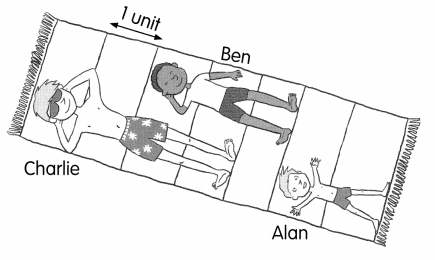Question 5.
Who is the tallest? __________
Answer: Charlie is the tallest with length of 4 units.

Question 6.
Who is the shortest? __________
Answer: Alan is the shortest with length of 2 units.

Write the names of the girls in the boxes.

Question 7.
Tia is taller than Nora. Sue is the tallest.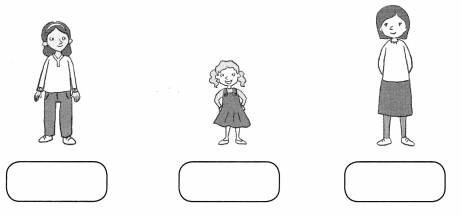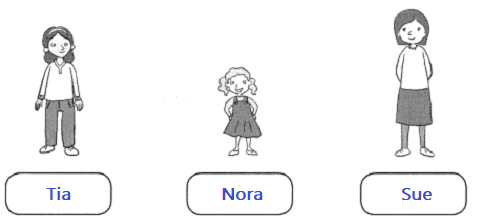Look at the picture and read. Then draw.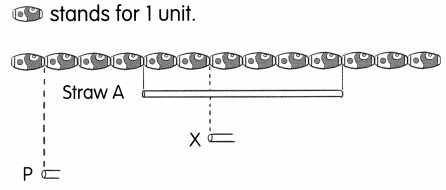Question 8.
Draw a straw as long as Straw A. Start at P.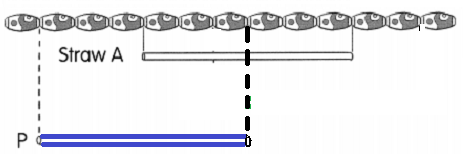Question 9.
Draw a straw longer than Straw A. Start at X.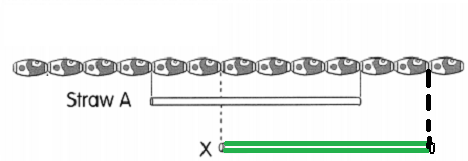Arrange the bears in order. Write the letter.

Question 10.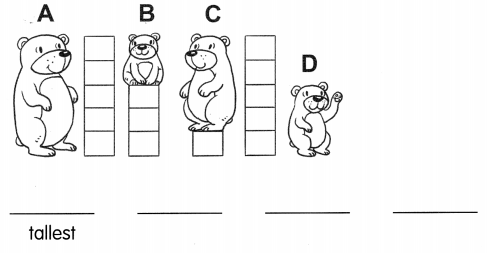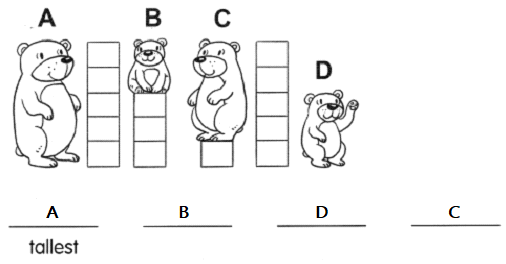Problem Solving

Fill in the blanks.

Question 1.
Tim, Ella, Rosa, and Ling knit some scarves. Who does each scarf belong to?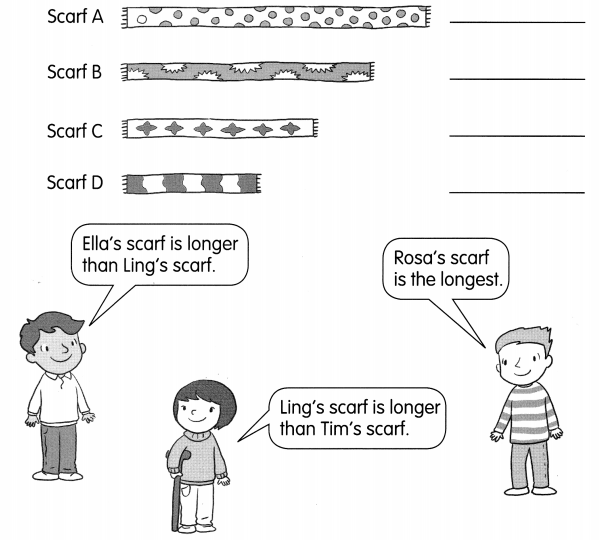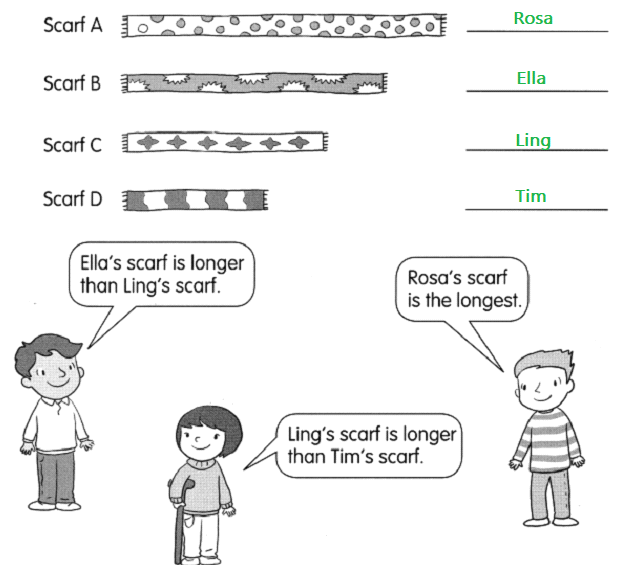Chapter Review/Test
Vocabulary
Match.

Question 1.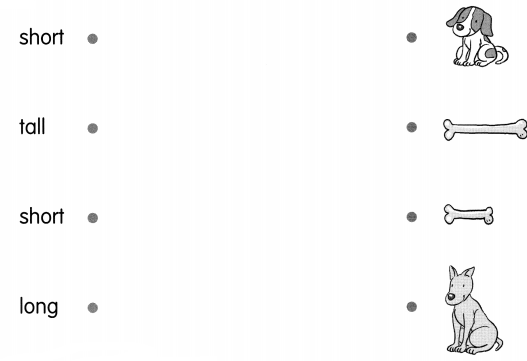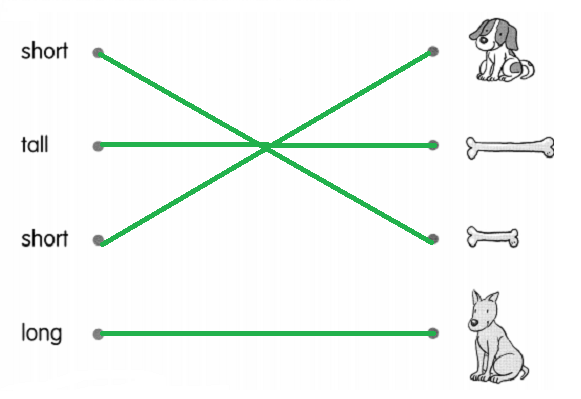Write longest or shortest.

Question 2.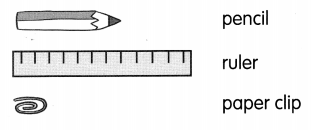The paper clip is the __________
The ruler is the __________
The paper clip is the shortest
The ruler is the longest

Concepts and Skills
Draw a start line.

Question 3.
Color the longest ribbon yellow. Color the shortest ribbon blue.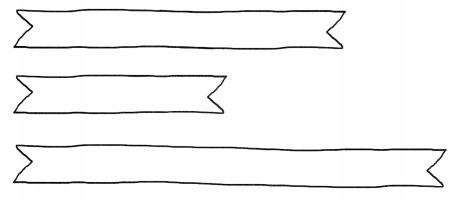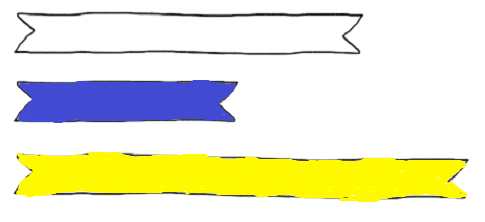Fill in the blanks.

Question 4.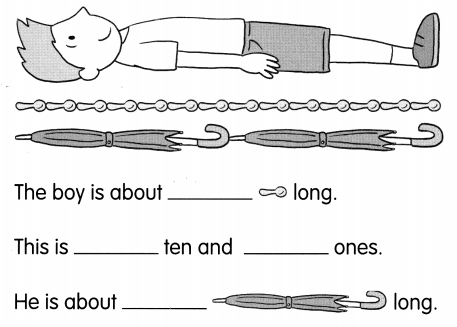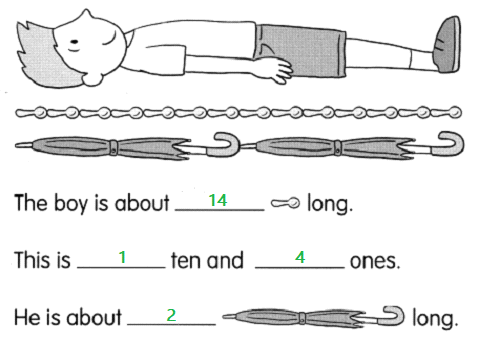Problem Solving

Solve.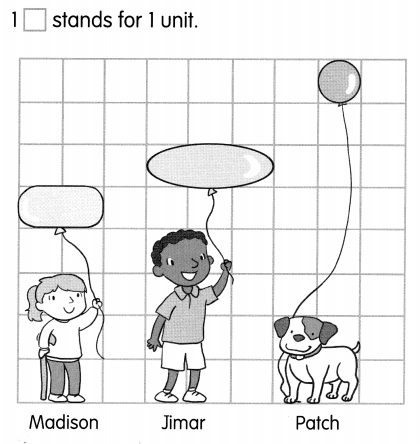Question 5.

Question 6.
Patch is ___ units tall and ___ units long.
Answer: Patch is 2 units tall and 2 units long.

Question 7.
The longest balloon is ____ units long.
Answer: The longest balloon is 3 units long.

Question 8.
Whose balloon has the longest string? __________
Answer: Patch’s balloon has the longest string.

Solve.

Question 9.
Three children are on stage. Ben is taller than Ally. Charlie is shorter than Ben. Ally is the shortest. Who is the tallest? _____ is the tallest. Who is not the shortest and not the tallest?